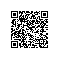# 数据结构和算法17 之拓扑排序

这一节我们学习一个新的排序算法，准确的来说，应该叫“有向图的拓扑排序”。所谓有向图，就是A->B，但是B不能到A。与无向图的区别是，它的边在邻接矩阵里只有一项（友情提示：如果对图这种数据结构部不太了解的话，可以先看一下这篇博文：数据结构和算法之 无向图。因为拓扑排序是基于图这种数据结构的）。

 A B C A 0 1 1 B 0 0 1 C 0 0 0

所以针对前面讨论的无向图，邻接矩阵的上下三角是对称的，有一半信息是冗余的。而有向图的邻接矩阵中所有行列之都包含必要的信息，它的上下三角不是对称的。所以对于有向图，增加边的方法只需要一条语句：

1. //有向图中，邻接矩阵中只有一项
2. public void addEdge(int start, int end) {
4. }
如果使用邻接表示意图，那么A->B表示A在它的链表中有B，但是B的链表中不包含A，这里就不多说了，本文主要通过邻接矩阵实现。

因为图是有向的，假设A->B->C->D这种，那这就隐藏了一种顺序，即要想到D，必须先过C，必须先过B，必须先过A。它们无形中形成了一种顺序，这种顺序在实际中还是用的挺广泛的，比如，要做web开发，必须先学Java基础等等，这些都遵循一个顺序，所以拓扑排序的思想也是这样，利用有向图特定的顺序进行排序。但是拓扑排序的结果不是唯一的，比如A->B的同时，C->B，也就是说A和C都能到B，所以用算法生成一个拓扑排序时，使用的方法和代码的细节决定了会产生那种拓扑排序。

拓扑排序的思想虽然不寻常，但是却很简单，有两个必要的步骤：

1. 找到一个没有后继的顶点；

2.从图中删除这个顶点，在列表中插入顶点的标记

然后重复1和2，直到所有顶点都从图中删除，这时候列表显示的顶点顺序就是拓扑排序的结果了。

但是我们需要考虑一种特殊的有向图：环。即A->B->C->D->A。这种必然会导致找不着“没有后继的节点”，这样便无法使用拓扑排序了。

下面我们分析下拓扑排序的代码：

1. public void poto() {
2.     int orig_nVerts = nVerts; //记录有多少个顶点
3.     while(nVerts > 0) {
4.         //返回没有后继顶点的顶点
5.         int currentVertex = noSuccessors(); //如果不存在这样的顶点，返回-1
6.         if(currentVertex == -1) {
7.             System.out.println("ERROR: Graph has cycles!");
8.             return;
9.         }
10.
11.         //sortedArray中存储排过序的顶点（从尾开始存）
12.         sortedArray[nVerts-1] = vertexArray[currentVertex].label;
13.         deleteVertex(currentVertex);//删除该顶点，便于下一次循环，寻找下一个没有后继顶点的顶点
14.     }
15.     System.out.println("Topologically sorted order:");
16.     for(int i = 0; i < orig_nVerts; i++) {
17.         System.out.print(sortedArray[i]);
18.     }
19.     System.out.println("");
20. }
主要的工作在while循环中进行，这个循环直到定点数为0时才退出：
1. 调用noSuccessors()找到任意一个没有后继的顶点；

2. 如果找到一个这样的顶点，把顶点放到sortedArray数组中，并且从图中删除这个顶点；

3. 如果不存在这样的顶点，则图必然存在环。

最后sortedArray数组中存储的就是排过序的顶点了。下面我们分析下noSuccessor()方法和deleteVertes()方法：

1. //return vertex with no successors
2. private int noSuccessors() {
3.     boolean isEdge;
4.     for(int row = 0; row < nVerts; row++) {
5.         isEdge = false;
6.         for(int col = 0; col < nVerts; col++) {
8.                 isEdge = true;
9.                 break;
10.             }
11.         }
12.         if(!isEdge) {//只要有边，返回最后一个顶点
13.             return row;
14.         }
15.     }
16.     return -1;
17. }
18.
19. private void deleteVertex(int delVertex) {
20.     if(delVertex != nVerts -1) {
21.         for(int i = delVertex; i < nVerts-1; i++) { //delete from vertexArray
22.             vertexArray[i] = vertexArray[i+1];
23.         }
25.         for(int row = delVertex; row < nVerts-1; row++) {//delete row from adjMat
26.             moveRowUp(row, nVerts);
27.         }
28.
29.         for(int col = delVertex; col < nVerts-1; col++) {//delete column from adjMat
30.             moveColLeft(col, nVerts-1);
31.         }
32.     }
33.     nVerts--;
34. }

1. private void moveRowUp(int row, int length) {
2.     for(int col = 0; col < length; col++) {
4.     }
5. }
6.
7. private void moveColLeft(int col, int length) {
8.     for(int row = 0; row < length; row++) {
10.     }
11. }
这样便介绍完了拓扑排序的所有过程了。下面附上完整的代码：

1. package graph;
2. /**
3.  * 有向图的拓扑排序：
4.  * 拓扑排序是可以用图模拟的另一种操作，它可以用于表示一种情况，即某些项目或事件必须按特定的顺序排列或发生。
5.  * 有向图和无向图的区别是：有向图的边在邻接矩阵中只有一项。
6.  * 拓扑排序算法的思想虽然不寻常但是很简单，有两个步骤是必须的：
7.  * 1. 找到一个没有后继的顶点
8.  * 2. 从图中删除这个顶点，在列表的前面插入顶点的标记
9.  * 重复这两个步骤，直到所有顶点都从图中删除，这时，列表显示的顶点顺序就是拓扑排序的结果。
10.  * 删除顶点似乎是一个极端的步骤，但是它是算法的核心，如果第一个顶点不处理，算法就不能计算出要处理的第二个顶点。
11.  * 如果需要，可以再其他地方存储图的数据(顶点列表或者邻接矩阵)，然后在排序完成后恢复它们。
12.  * @author eson_15
13.  * @date 2016-4-20 12:16:11
14.  *
15.  */
16. public class TopoSorted {
17.     private final int MAX_VERTS = 20;
18.     private Vertex vertexArray[]; //存储顶点的数组
19.     private int adjMat[][]; //存储是否有边界的矩阵数组, 0表示没有边界，1表示有边界
20.     private int nVerts; //顶点个数
21.     private char sortedArray[]; //存储排过序的数据的数组
22.
23.     public TopoSorted() {
24.         vertexArray = new Vertex[MAX_VERTS];
26.         nVerts = 0;
27.         for(int i = 0; i < MAX_VERTS; i++) {
28.             for(int j = 0; j < MAX_VERTS; j++) {
30.             }
31.         }
32.         sortedArray = new char[MAX_VERTS];
33.     }
34.
35.     public void addVertex(char lab) {
36.         vertexArray[nVerts++] = new Vertex(lab);
37.     }
38.
39.     //有向图中，邻接矩阵中只有一项
40.     public void addEdge(int start, int end) {
42.     }
43.
44.     public void displayVertex(int v) {
45.         System.out.print(vertexArray[v].label);
46.     }
47.
48.     /*
49.      * 拓扑排序
50.      */
51.     public void poto() {
52.         int orig_nVerts = nVerts; //remember how many verts
53.         while(nVerts > 0) {
54.             //get a vertex with no successors or -1
55.             int currentVertex = noSuccessors();
56.             if(currentVertex == -1) {
57.                 System.out.println("ERROR: Graph has cycles!");
58.                 return;
59.             }
60.
61.             //insert vertex label in sortedArray (start at end)
62.             sortedArray[nVerts-1] = vertexArray[currentVertex].label;
63.             deleteVertex(currentVertex);
64.         }
65.         System.out.println("Topologically sorted order:");
66.         for(int i = 0; i < orig_nVerts; i++) {
67.             System.out.print(sortedArray[i]);
68.         }
69.         System.out.println("");
70.     }
71.
72.     //return vertex with no successors
73.     private int noSuccessors() {
74.         boolean isEdge;
75.         for(int row = 0; row < nVerts; row++) {
76.             isEdge = false;
77.             for(int col = 0; col < nVerts; col++) {
79.                     isEdge = true;
80.                     break;
81.                 }
82.             }
83.             if(!isEdge) {
84.                 return row;
85.             }
86.         }
87.         return -1;
88.     }
89.
90.     private void deleteVertex(int delVertex) {
91.         if(delVertex != nVerts -1) {
92.             for(int i = delVertex; i < nVerts-1; i++) { //delete from vertexArray
93.                 vertexArray[i] = vertexArray[i+1];
94.             }
95.
96.             for(int row = delVertex; row < nVerts-1; row++) {//delete row from adjMat
97.                 moveRowUp(row, nVerts);
98.             }
99.
100.             for(int col = delVertex; col < nVerts-1; col++) {//delete column from adjMat
101.                 moveColLeft(col, nVerts-1);
102.             }
103.         }
104.         nVerts--;
105.     }
106.
107.     private void moveRowUp(int row, int length) {
108.         for(int col = 0; col < length; col++) {
110.         }
111.     }
112.
113.     private void moveColLeft(int col, int length) {
114.         for(int row = 0; row < length; row++) {
116.         }
117.     }
118. }

拓扑排序就介绍到这吧，如有错误之处，欢迎留言指正~使用钉钉扫一扫加入圈子
+ 订阅